Question
Find the electric field at a point P due to a 10 × 10^−9C charged disk of radius 2cm. The charges are fixed and uniformly distributed on the disk. The point P is 33 cm away from the disk. Now you drill a hole of radius 0.35cm at the center of the disk. Find the electric field of this new ”disk” without taking the difference between the two disks, rather solve by integration from the small to bigger radius.

It is well known that the electric field at a perpendicular distance z from the origin of a circular wire with charge Q and radius r is given by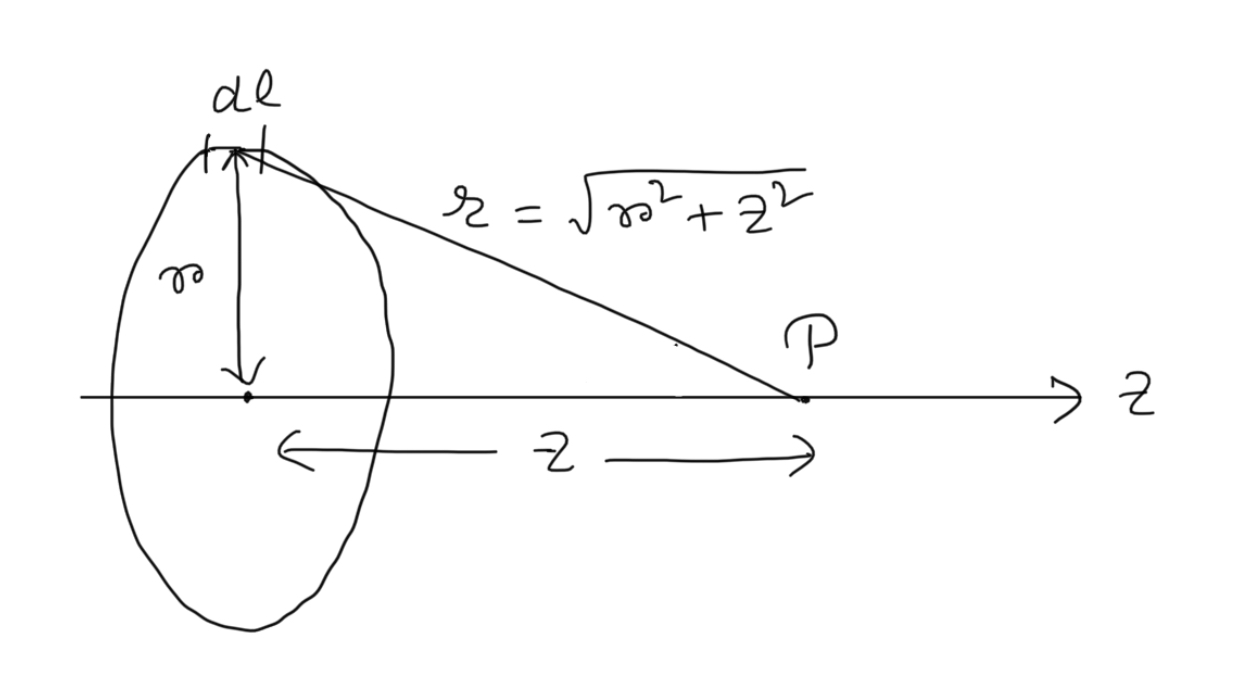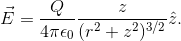Hence the electric field at a perpendicular distance z from the origin of a circular disk with charge Q and radius R is given by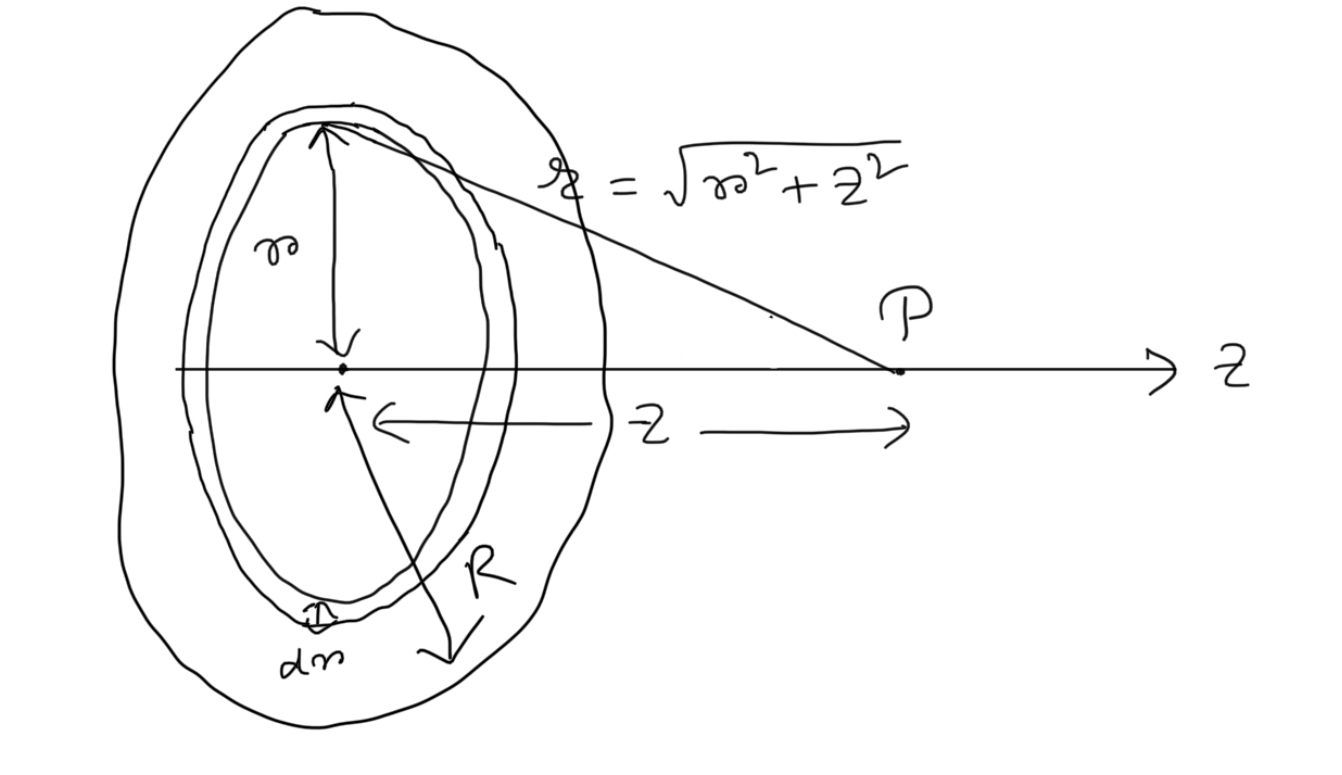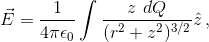where dQ  is the charge of the elementary ring of radius r and thickness dr.

Now, in this case,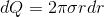, where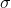is the surface charge density of the disk , i.e.,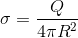.

Hence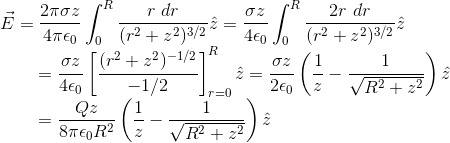.

Here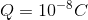,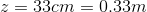,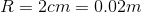and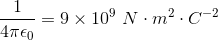.

Hence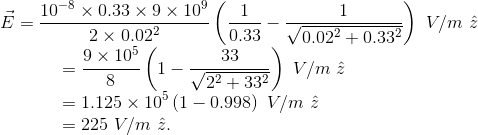After drilling the hole of radius R0=0.35 cm, the electric field becomes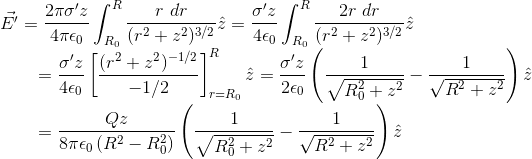since in this case, the area of the disk is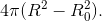Hence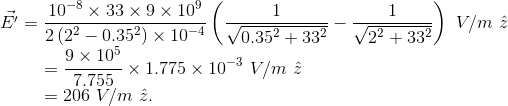#### Earn Coins

Coins can be redeemed for fabulous gifts.

Similar Homework Help Questions
• ### The total electric field at a point on the axis of a uniformly charged disk, which...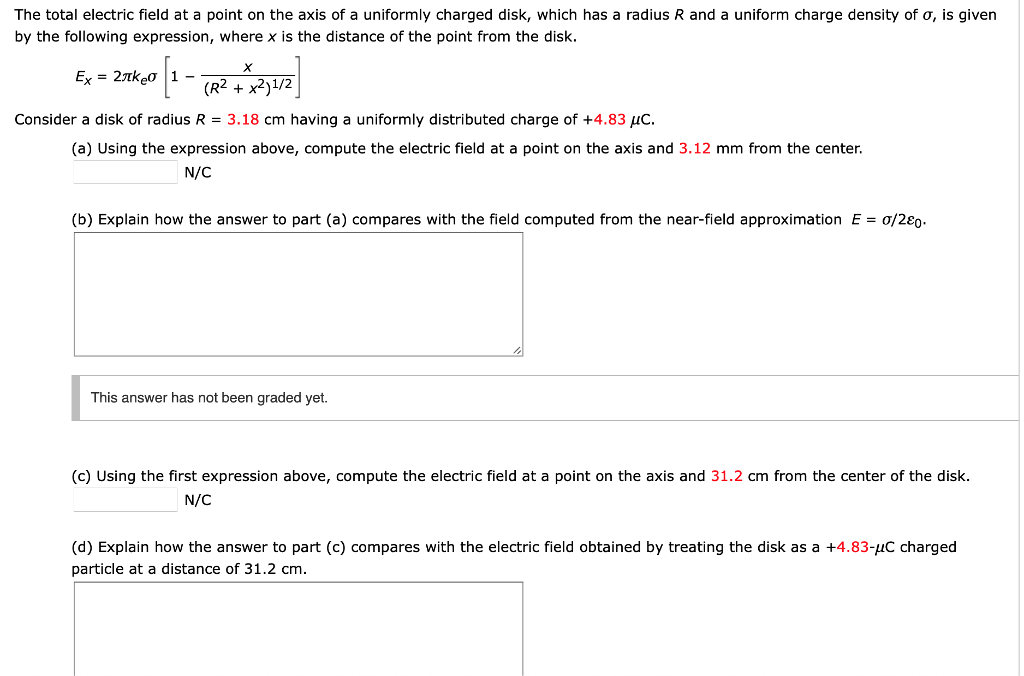The total electric field at a point on the axis of a uniformly charged disk, which has a radius R and a uniform charge density of σ, is given by the following expression, where x is the distance of the point from the disk. (R2 + x2)1/2 Consider a disk of radius R-3.18 cm having a uniformly distributed charge of +4.83 C. (a) Using the expression above, compute the electric field at a point on the axis and 3.12 mm...

• ### The total electric field at a point on the axis of a uniformly charged disk, which...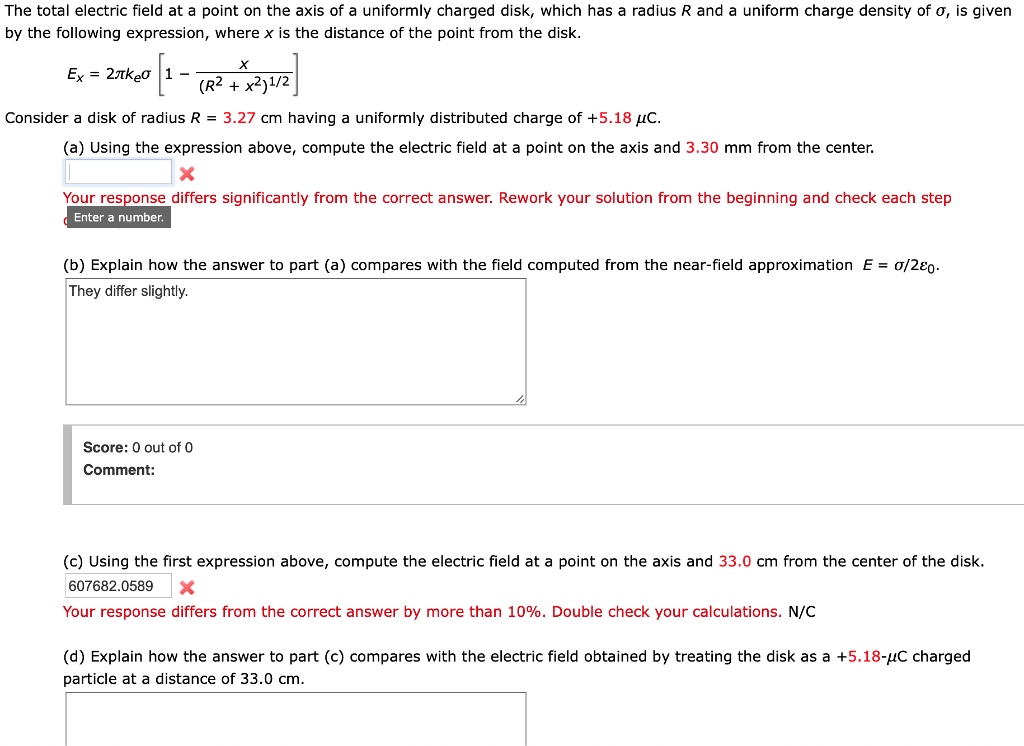The total electric field at a point on the axis of a uniformly charged disk, which has a radius R and a uniform charge density of σ, is given by the following expression, where x is the distance of the point from the disk. (R2 + x2)1/2 Consider a disk of radius R-3.27 cm having a uniformly distributed charge of +5.18 C. (a) Using the expression above, compute the electric field at a point on the axis and 3.30 mm...

• ### Find the electric field due to a disk at point X, L distance away. Integrate using...

Find the electric field due to a disk at point X, L distance away. Integrate using a ring of charge r distance away from the center. R - Radius - Charge/unit area

• ### Please explain and solve 3 Apl 2019 04) (25 points) The figure shows a non-conducting (thin) disk with a hole. The radius of the disk is Ri and the radius of the hole is R1. A total charge Q is un...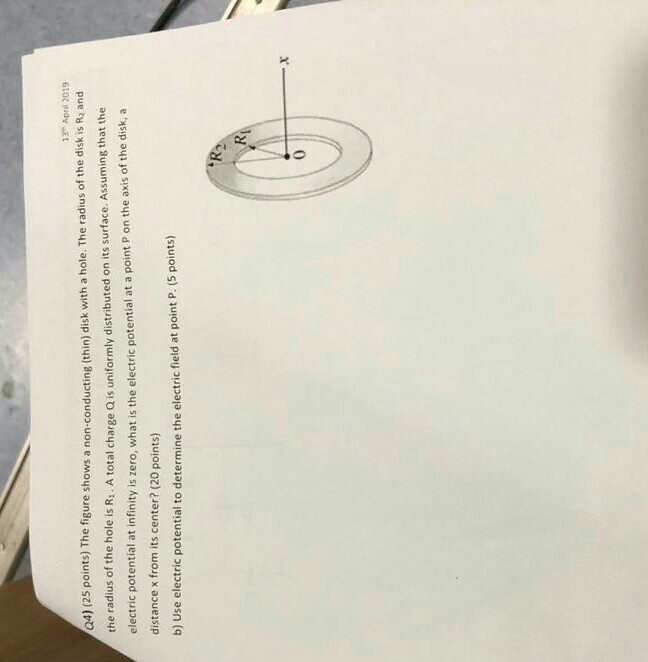Please explain and solve 3 Apl 2019 04) (25 points) The figure shows a non-conducting (thin) disk with a hole. The radius of the disk is Ri and the radius of the hole is R1. A total charge Q is uniformly distributed on its surface electric potential at infinity is zero, what is the el distance x from its center? (20 points) b) Use electric potential to determine the electric field at point P. (S points) . Assuming that the...

• ### What is the electric field due to a ring of charge Q at a point P...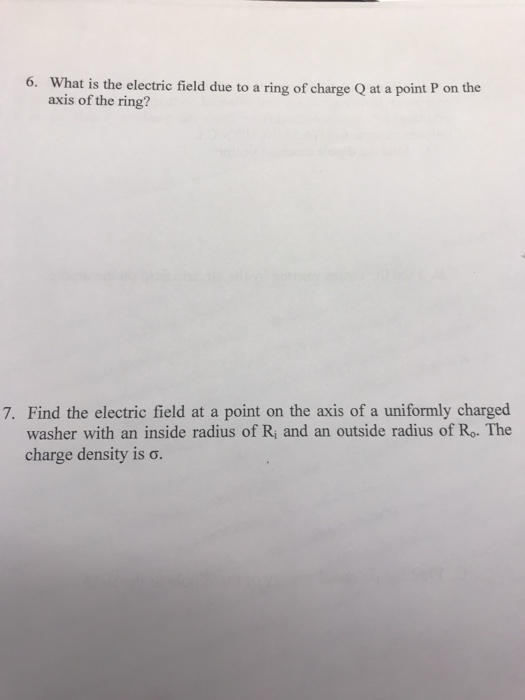What is the electric field due to a ring of charge Q at a point P on the axis of the ring? 6. 7. Find the electric field at a point on the axis of a uniformly charged washer with an inside radius of Ri and an outside radius of Ro. The charge density is σ.

• ### Tipler6 23.P.085. Along the axis of a uniformly charged disk, at a point 0.6 m from...

Tipler6 23.P.085. Along the axis of a uniformly charged disk, at a point 0.6 m from the center of the disk, the potential is 56.9 V and the magnitude of the electric field is 63.2 V/m; at a distance of 1.5 m, the potential is 27.1 V and the magnitude of the electric field is 16.5 V/m. Find the total charge residing on the disk.

• ### Tipler6 23.P.085. Along the axis of a uniformly charged disk, at a point 0.6 m from...

Tipler6 23.P.085. Along the axis of a uniformly charged disk, at a point 0.6 m from the center of the disk, the potential is 56.9 V and the magnitude of the electric field is 63.2 V/m; at a distance of 1.5 m, the potential is 27.1 V and the magnitude of the electric field is 16.5 V/m. Find the total charge residing on the disk.

• ### Suppose you design an apparatus in which a uniformly charged disk of radius R is to produce an electric field.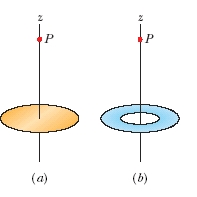Suppose you design an apparatus in which a uniformly charged disk of radius R is to produce an electric field. The field magnitude is most important along the central perpendicular axis of the disk, at a point P at distance 4.60R from the disk (see Figure (a)). Cost analysis suggests that you switch to a ring of the same outer radius R but with inner radius R/4.60 (see Figure (b)). Assume that the ring will have the same surface charge...

• ### Suppose you design an apparatus in which a uniformly charged disk of radius R is to produce an electric field.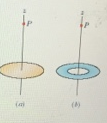Suppose you design an apparatus in which a uniformly charged disk of radius R is to produce an electric field. The field magnitude is most important along the central perpendicular axis of the disk, at a point P at distance 2.00R from the dis (see Figure (a)). Cost analysis suggests that you switch to a ring of the same outer radius R but with inner radius R/2.00 (see Figure (b)). Assume that the ring will have the same surface charge...